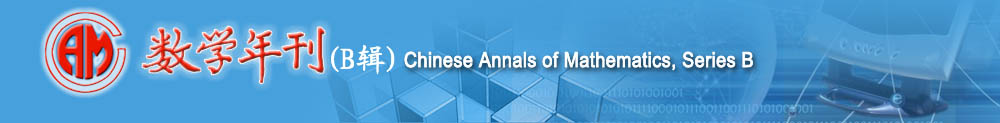On the Cegrell Classes Associated to a Positive Closed Current Citation： Mohamed ZAWAY.On the Cegrell Classes Associated to a Positive Closed Current[J].Chinese Annals of Mathematics B,2019,40(4):567~584 Page view： 936        Net amount： 773 Authors： Mohamed ZAWAY; Abstract： The aim of this paper is to study the operator $(dd^c\centerdot)^q\wedge T$ on some classes of plurisubharmonic (psh) functions, which are not necessary bounded, where $T$ is a positive closed current of bidimension $(q,q)$ on an open set $\Omega$ of $\Bbb C^n$. The author introduces two classes $\mathcal{F}_{p}^{T}(\Omega)$ and $\mathcal{E}_p^T(\Omega)$ and shows first that they belong to the domain of definition of the operator $(dd^c\centerdot)^q\wedge T$. Then the author proves that all functions that belong to these classes are $C_T$-quasi-continuous and that the comparison principle is valid for them. Keywords： Positive closed current, Plurisubharmonic function, Capacity,Monge-Amp`ere Operator Classification： 32U40, 32U05, 32U20 Download PDF Full-Text